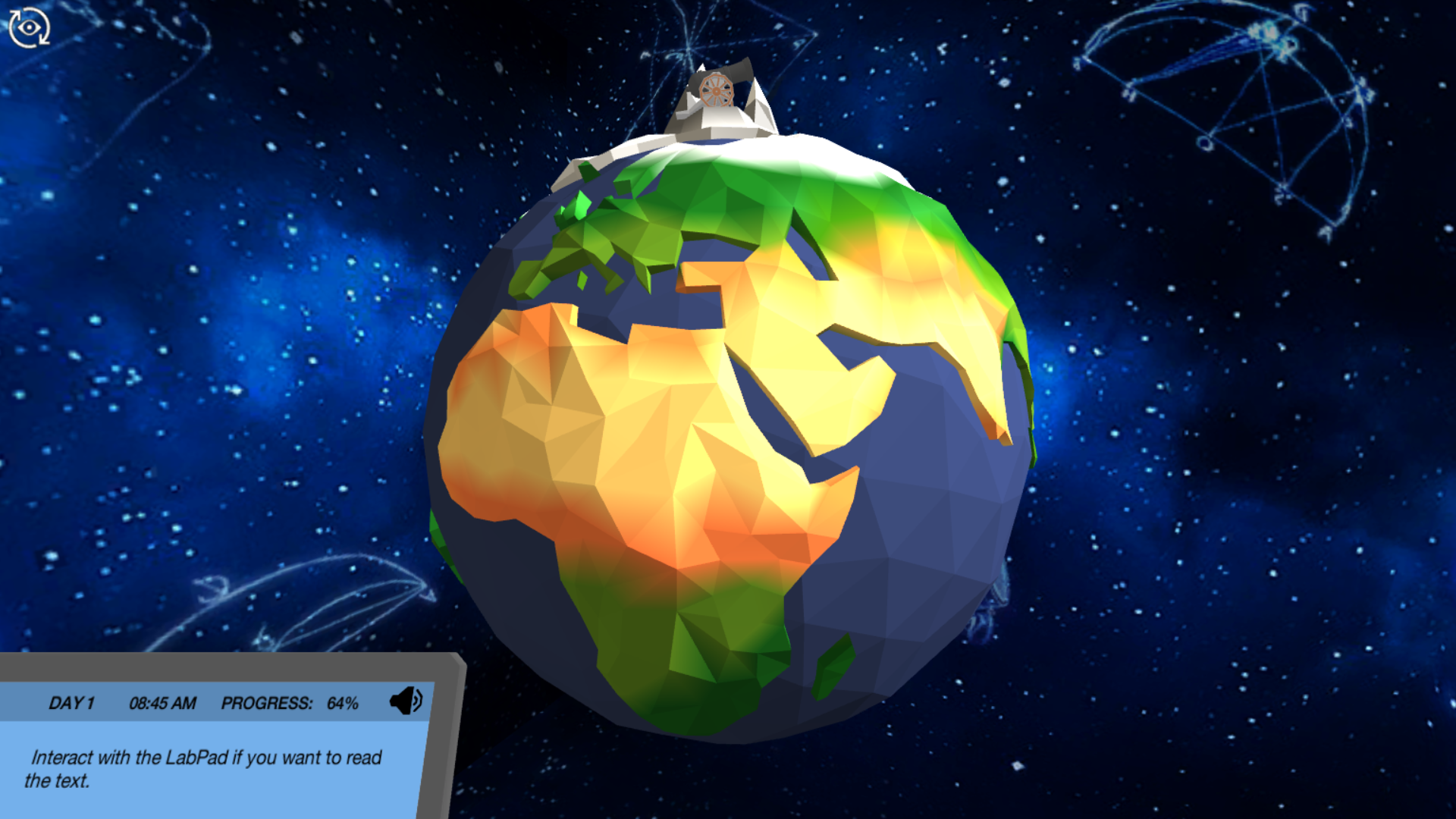# Law of Universal Gravitation: Use gravity to orbit the moon Virtual Lab

Learn the basics of gravitation by weighing the famous scientist Sir Isaac Newton and observe the Law of Universal Gravitation in action by changing the mass of the Earth. Travel in space and perform a thought experiment about orbits.Try our lab safety simulation

Discover one of our 200+ learning simulations today

• Description
• Features

## About the Law of Universal Gravitation: Use gravity to orbit the moon Virtual Lab

Can you imagine having a better teacher in the Law of Universal Gravitation than Sir Isaac Newton? In this simulation, you will learn about the difference between mass and weight. Newton himself will get on the scales and you will perform a pendulum experiment which will let you define the gravitational acceleration near the surface of the Earth. In order to deduce Newton’s Law of Gravitation, you will investigate how gravitational acceleration is dependent on masses of objects and the distance in between them. In your first mission, you will change the mass of the Earth and determine the “mass dependence” of the gravitational acceleration. But watch out! With great power in this initial mission comes great responsibility during your last mission…

Perform a thought experiment

Enter the mind of Newton and learn why the moon does not just crash onto the surface of the Earth. Observe how the initial tangential velocity affects the orbit of a cannon ball around the Earth and realize the “distance dependence” of the gravitational acceleration. You are now one step closer to guiding a spacecraft with astronauts on their mission to orbit the moon.

The solar system

In order to orbit the moon you have to know everything about the theory of gravity. In this simulation, you can learn all about it by having a look at the holo-table, where you can see the solar system orbit around the sun right in front of you. You will also learn the basic properties of the known planets of our solar system.

Orbit the moon

Changing the mass of the Earth during your first mission will affect the preset velocity of a spacecraft for entering a lunar orbit. Will you be able to find the correct velocity for entering circular orbit around the moon by changing the trajectories of the orbiting spacecraft?

Get started now

Learn the basics of gravitation by weighing the famous scientist Sir Isaac Newton and observe the Law of Universal Gravitation in action by changing the mass of the Earth. Travel in space and perform a thought experiment about orbits.

Techniques in lab
Thought experiment, Pendulum
Learning objectives

At the end of this simulation, you will be able to...

• Understand the difference between weight and mass
• Measure gravitational acceleration near the Earth's surface
• Describe the mechanism for circular orbits
• Describe the distance dependence of g
• Distinguish between bound and unbound trajectories
Simulation features

Length: 27 Minutes
Accessibility mode: Not available
Languages: English (United States), German, Spanish, French, Italian

NGSS

HS-PS2-4, HS-ESS1-4

IB

2.2 – Forces

6.1 – Circular motion

6.2 – Newton’s law of gravitation

AP

3.8 Applications of Circular Motion and Gravitation

3.4 Gravitational Field/Acceleration Due to Gravity on Different Planets

6.1 Period of Simple Harmonic Oscillators

2.2 The Gravitational Field

3.3 Gravitational and Electric Forces